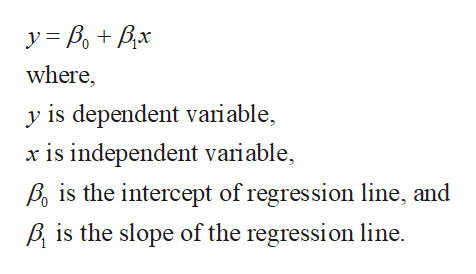# The regression equation relating attitude rating(x) and job performance (y) for ten randomly selected employees of a company is y^=117-1.02x. Predict the job performance rating for an employee whose attitude rating is 55. Show work.

Question
47 views

The regression equation relating attitude rating(x) and job performance (y) for ten randomly selected employees of a company is y^=117-1.02x. Predict the job performance rating for an employee whose attitude rating is 55. Show work.

check_circle

Step 1

Regression equation:

The regression tells about the relationship of two variables, one dependent ...help_outlineImage Transcriptionclosewhere, y is dependent variable, x is independent variable Po is the intercept of regression line, and is the slope of the regression line. fullscreen

### Want to see the full answer?

See Solution

#### Want to see this answer and more?

Solutions are written by subject experts who are available 24/7. Questions are typically answered within 1 hour.*

See Solution
*Response times may vary by subject and question.
Tagged in

### Other